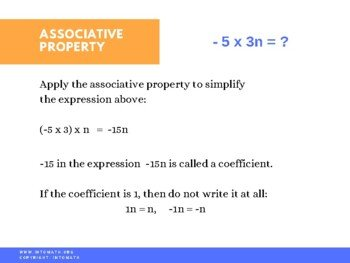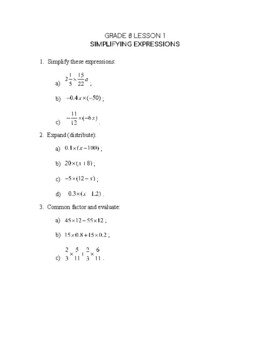CA$2.00 In this lesson you will learn about multiplication properties and their significance. For example, that a × b = b × a or that a(b + c) = ab + bc. You will learn how distributive property is used to simplify algebraic expressions. This property is also often used to solve equations. For example, when solving an equation 2(3x – 1) = 10 the first step would be to expand the expression with brackets. It would become 6x – 2 = 10. Then the equation could be solved using inverse operations and reverse BEDMAS. The lesson covers simplifying algebraic expressions by collecting like terms.Grade 8 Workbook (18 pages with answers) CA$4.00

Algebraic expressions are symbols or  combinations of symbols used in algebra, containing one or more numbers, variables, and arithmetic operations.

Like terms are terms that have the same variables with the same exponents or no variables – constants (4n and 5n, 6 and 89).